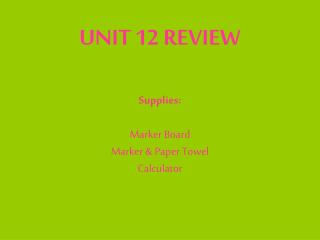DownloadDownload PresentationUNIT 12 REVIEW Supplies: Marker Board Marker & Paper Towel Calculator

# UNIT 12 REVIEW Supplies: Marker Board Marker & Paper Towel Calculator

Download Presentation## UNIT 12 REVIEW Supplies: Marker Board Marker & Paper Towel Calculator

- - - - - - - - - - - - - - - - - - - - - - - - - - - E N D - - - - - - - - - - - - - - - - - - - - - - - - - - -
##### Presentation Transcript

1. UNIT 12 REVIEWSupplies:Marker BoardMarker & Paper TowelCalculator

2. Write the equilibrium expression: 4 HCl(g) + O2(g)   2 Cl2(g) + 2 H2O(g) ANSWER: Keq = [Cl2]2 [H2O]2 [HCl]4 [O2]

3. Write the equilibrium expression: NCl3(g) + Cl2(g)   NCl5(g) Answer: Keq = [NCl5] [Cl2] [NCl3]

4. Write the equilibrium expression 2 NO(g) + O2(g)   2 NO2(g) Answer: Keq = [NO2]2 [NO]2 [O2]

5. What 2 types of changes cause a reaction to go to completion? • If a reaction does not go to completion, what may happen to some of the products? • Answers: • Forms a Gas (g) or a Precipitate ( ) • Can reform reactants (Reverse Reaction)

6. A reaction that does not go to completion is called a __________________ reaction. • What part of the equation shows if a reaction goes to completion or not? • Answer: • Reversible • the arrow • (double = reversible, single = completion)

7. When a system is at ________________________ , it will stay that way until something changes. • State Le Chatelier’s Principle. • List three examples of stresses that can change a system at equilibrium. • 1) • 2) • 3) • Answers: • Equilibrium • When a reaction at equilibrium is disturbed it will shift to eliminate the stress • Changing temperature, concentration, or pressure/volume (only for gases)

8. You can tell if a reaction is endo- or exothermic by looking at the equation. If heat is on the reactant side the reaction is endothermic. If heat is on the product side, the reaction is exothermic. Determine if these reactions are endothermic or exothermic. • a) 2NO2 ↔ N2O4 + 58.8 J b) AgCl + energy ↔ Ag + Cl • _____________________ ________________________ • What will happen if the temperature in a) is raised? ________________________ • How could you make reaction “b” produce more products? Add energy or remove energy???? • Answers: a) exothermic b) endothermic • Shift left • Add energy

9. When an equilibrium mixture is subjected to a decreased pressure, it will shift to the side with ____________________. Answer: Most Moles Decrease Pres = Vol Increase = most moles

10. CH4(g) + 2 O2(g)   CO2(g) + 2 H2O(g) • Calculate Keq if: [CH4] = 0.0150M, [O2] = 0.00235 M, [CO2] = 0.0824M, [H2O]= 0.0603M • Are the reactants or products favored at equilibrium? Answer: Keq = [CO2] [H2O]2 [CH4] [O2]2 Keq = (0.0824)(0.0603)2 = 3,620 (0.0150)(0.00235)2 Products are favored.

11. Calculate Keq • PCl5(g)   PCl3(g) + Cl2(g) • [PCl5] = 1.00M, [PCl3]=3.16x10-2M, [Cl2]=7.18x10-3M • Are the reactants or products favored at equilibrium? • If you change the temperature, would the Keq be the same? Answers: Keq = [PCl3][Cl2] = (3.16x10-2)(7.18x10-3) = 2.27x10-4 [PCl5] (1.00) Reactants are favored. No.

12. Why does the word dynamic describe equilibrium. • Define reversible reaction. • Define completion reaction. Answers: Dynamic describes equilibrium because the reaction does not stop. The reactions continue in the forward and reverse reactions. A reversible reaction can form products and then reform reactants. A completion reaction can only form products. Forms a gas or a precipitate.

13. Write the equilibrium expression • Calculate Keq CO(g) + 2H2(g)  CH3OH(g) [CO]=0.025M, [H2]=0.080M, [CH3OH]=0.063M Answers:[CH3OH] = (0.063) = 394 [CO] [H2]2 (0.025)(0.0802)

14. What does Ksp stand for? • What does it describe? • Which lead compound below is the least soluble? • Ksp = PbBr2 6.3 x 10-6 • Ksp = PbCl2 1.7 x 10-5 • Ksp = PbCrO4 1.8 x 10-14 • Answers: • Solubility Product Constant • Ksp describes the solubility (ability to dissolve) of slightly soluble substances. • PbCrO4 because it has the smallest Ksp